# LSMESTIMATE Statement

This statement documentation applies to the following procedures:
GENMOD, LOGISTIC, MIXED, ORTHOREG, PHREG, PLM, SURVEYLOGISTIC, SURVEYPHREG, and SURVEYREG. The LSMESTIMATE statement in the GLIMMIX procedure is documented in Chapter 40, The GLIMMIX Procedure.

The LSMESTIMATE statement provides a mechanism for obtaining custom hypothesis tests among least squares means. In contrast to the LSMEANS statement, the LSMESTIMATE statement does not produce the least squares means or their differences; instead, you can estimate any linear function of the least squares means (including the means themselves or their differences). In contrast to the linear functions that are constructed with the ESTIMATE statement, you do not specify coefficients for the individual parameter estimates. Instead, with the LSMESTIMATE statement you specify coefficients for the least squares means; these are then converted for you into estimable functions for the parameter estimates.

The LSMESTIMATE statement thus combines important and convenient features of the LSMEANS and the ESTIMATE statement. As with the LSMEANS statement, the following conditions are true:

• You need to specify only a single effect; the mapping into linear estimable functions in terms of the parameter estimates is performed by the procedure.

• You can use the AT=, BYLEVEL, and OBSMARGINS options to affect the computation of the underlying least squares means.

As with the ESTIMATE statement you can do the following:

• specify multiple-row linear combinations.

• perform multiplicity corrections to control the familywise Type I error probability with the ADJUST= option.

• construct general linear functions of the least squares means.

• perform joint F or chi-square tests with or without order restrictions through the JOINT option.

• rely on positional or nonpositional syntax to specify coefficients for linear functions. For details about using nonpositional syntax, see the section Positional and Nonpositional Syntax for Coefficients in Linear Functions.

The computation of an LSMESTIMATE involves two coefficient matrices. Suppose that there are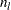levels for a valid least squares means effect (an effect that is part of your model and consists of classification variables only). Then the LS-means are formed as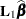, whereis a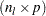coefficient matrix. The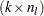coefficient matrix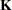is formed from the values that you supply in therows of the LSMESTIMATE statement. The least squares means estimates then represent the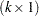vector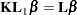Because the analytic features and capabilities of the LSMESTIMATE statement are an amalgam of the LSMEANS and the ESTIMATE statement, the syntax of the statement follows the same pattern.